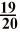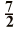# Learn Fraction Number#What do you mean by fraction in math?

An online fraction number definition

When an object is divided into a number of equal parts then each part is called a fraction.

Fraction can be expressible by the quotient of two numbers as in a/b, "b" greater than 1, where "a" is called the numerator and "b" is called the denominator.

### Proper Fraction

If "a" is smaller than "b" it is a proper fraction.

Example:### Improper Fraction

If "a" is greater than "b" or "a" is equal to "b" it is an improper fraction.

Example:### Math Number Symbols

R - Real Numbers
Z - Integers
N - Natural Numbers
Q - Rational Numbers
P - Irrational Numbers#Related Number Types

##Math number calculation ►

Online Algebra calculation, formulas , Digital calculation, Statistical calculation, Math Converters Pet Age Calculator